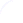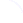Search
 Submission Procedure share: |available in:   PDF (294 kB) PS (530 kB)

get:
 Similar Docs BibTeX Write a comment
get:

### Using Place Invariants and Test Point Placement to Isolate Faults in Discrete Event Systems

Iwan Georgiew Tabakow (Wroclaw University of Technology, Poland)

Abstract: This paper describes a method of using Petri net P-invariants in system diagnosis. To model this process a net oriented fault classification is presented. Hence, the considered discrete event system is modelled by a live, bounded, and reversible place-transition Petri net. The notions of D-partition of the set of places P of a given place-transition net N and net k-distinguishability are first introduced. Next these two notions are extended to the set of all vertices, i.e. places and transitions of N. So the problem of fault identification of the vertices of N is transformed as a problem of fault identification of the places of a new net N´ called a net simulator of N. Any transition in N´ is assumed to be fault-free. Then the corresponding net place invariants are computed. The system k-distinguishability measure is obtained in a unique way from the place-invariant matrix. For a large value of k, the system model is extended by using some set of additional places called test points and at the same time preserving the original net properties. To obtain a 1-distinguishable net the notion of a marked graph component is used. It is shown a sufficient condition for 1-distinguishability of an arbitrary place-transition net and a corresponding algorithm is presented. Next two different diagnosis test strategies are discussed, i.e. combinational and sequential fault diagnosis. Corresponding (single) place and transition fault models are introduced. The complexity of the proposed method depends on the effectivity of the existing algorithms for computation of the P-cover, i.e. the set of P-invariants covering N. The proposed approach can be extended for higher level Petri nets, e.g such as coloured nets or also to design self-diagnosable circuit realisations of Boolean interpreted Petri nets. Several examples are given.

Keywords: D-partition, P-invariant, discrete event system, fault diagnosis, k-distinguishability, place-transition net, test point

Categories: C.3, D.2.2, G.2.3, H.1.1, J.6, J.7